Equal Groups
Number Line
Word Problems
Fast Facts
Arrays
100

What multiplication sentence does this show?2 x 3 = 6

100

What multiplication sentence does this show?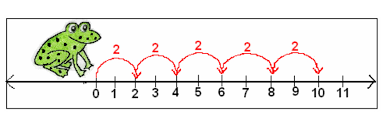5 x 2 = 10

100

Eddie has 2 bags of candy. Each bag has 10 pieces of candy. How much candy does Eddie have in all?

2 x 10 = 20

100
What is 4x0?

0

100

What multiplication equation does this show?3 x 2 = 6

200

What multiplication sentence does this show?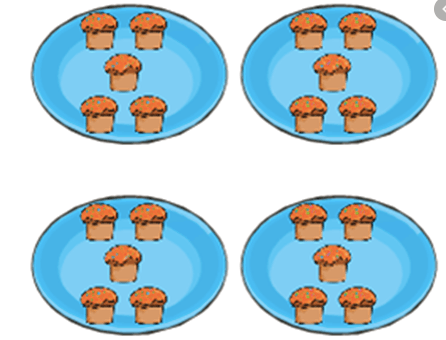4 x 5 = 20

200

What multiplication sentence does this show?2 x 3 = 6

200

Heilly has a sticker book. On one page, she has 3 rows of stickers with 5 stickers in each row. How many stickers does Heilly have in all?

3 x 5 = 15

200

What is 6 x 1?

6

200

What multiplication equation does this show?3 x 4 = 12

300

What multiplication sentence does this show?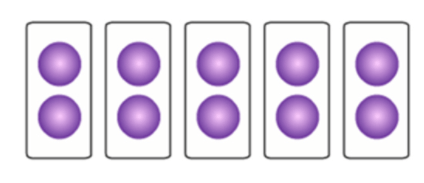5 x 2 = 10

300

What multiplication sentence does this show?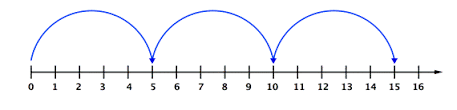3 x 5 = 15

300

Arlette has 5 plates of cookies. Each plate has 4 cookies on it. How many cookies does Arlette have in all?

5 x 4 = 20

300

What is 2 x 8?

16

300

What multiplication equation does this show?5 x 6 = 30
400

What multiplication sentence does this show?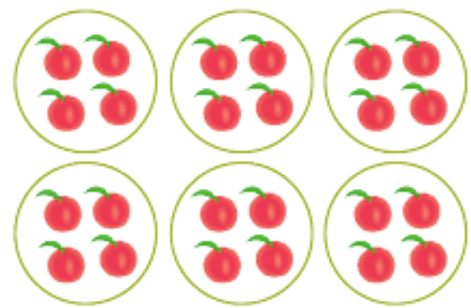6 x 4 = 24

400

What multiplication sentence does this show?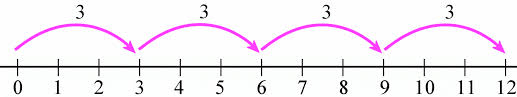4 x 3 = 12

400

Henry has a book shelf. There are 2 shelves with 7 books on each. How many books does Henry have in all?

2 x 7 = 14

400

What is 5 x 7?

35

400

What multiplication equation does this show?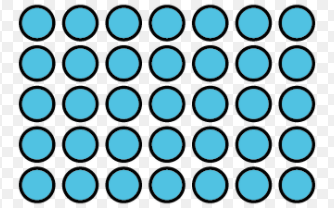5 x 7 =  35

500

What multiplication equation does this show?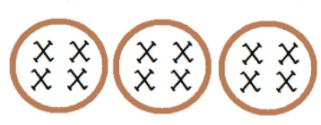3 x 4 = 12

500

What multiplication sentence does this show?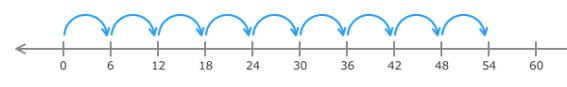9 x 6 = 54

500

Ms. Karlin made 9 bracelets. Each bracelet had 6 beads on it. How many beads in all did Ms. Karlin use to make her bracelets?

6 x 9 = 54

500

What is 9x9?

81

500

What multiplication equation does this show?3 x 6 = 18
Click to zoom